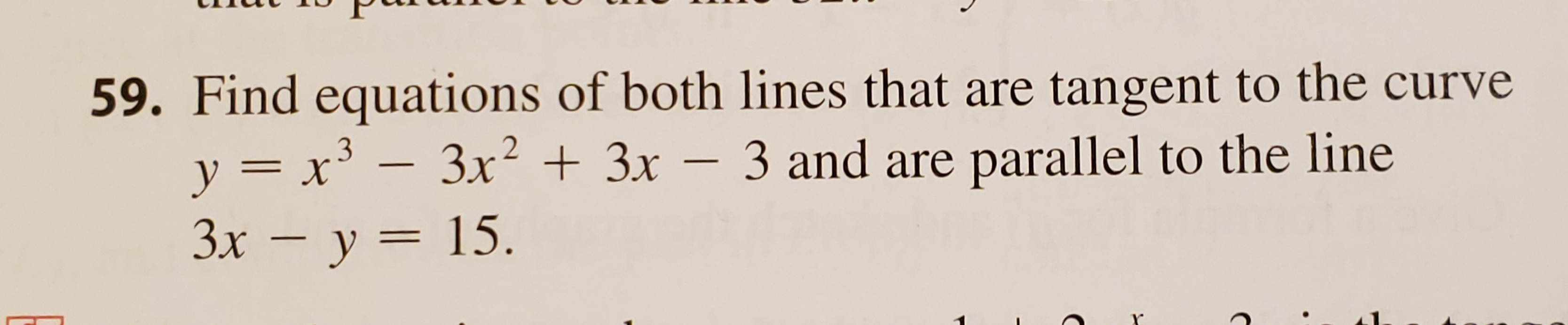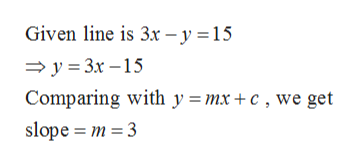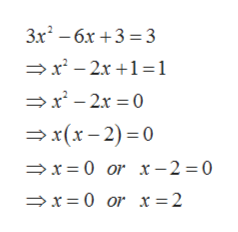# 59. Find equations of both lines that are tangent to the curve3x23x-3 and are parallel to the liney =x3Зх — у 3D 15.

Question
3 viewshelp_outlineImage Transcriptionclose59. Find equations of both lines that are tangent to the curve 3x23x-3 and are parallel to the line y =x3 Зх — у 3D 15. fullscreen
check_circle

Step 1

First we find the slope of the tangent:

Slope of the tangent is the derivative of the given curve.

Therefore differentiating given function with respect to x, we get

Step 2

Now we find the slope of the given line:help_outlineImage TranscriptioncloseGiven line is 3x -y = 15 y 3x -15 Comparing with y = mx +c , we get slope m 3 fullscreen
Step 3

Since tangents are parallel to the given line, therefore their slopes must ...help_outlineImage Transcriptionclose3x2 - 6x 3 3 x2-2x +1 1 >x'-2x x(x-2)0 x 0 or x-2 =0 x 0 or x =2 fullscreen

### Want to see the full answer?

See Solution

#### Want to see this answer and more?

Solutions are written by subject experts who are available 24/7. Questions are typically answered within 1 hour.*

See Solution
*Response times may vary by subject and question.
Tagged in

### Derivative